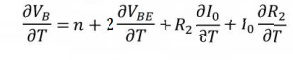Home | | Linear Integrated Circuits | Voltage References

# Voltage References

The circuit that is primarily designed for providing a constant voltage independent of changes in temperature is called a voltage reference.

Voltage References

The circuit that is primarily designed for providing a constant voltage independent of changes in temperature is called a voltage reference. The most important characteristic of a voltage reference is the temperature coefficient of the output= reference voltage TcR , and it is expressed asThe desirable properties of a voltage reference are:

1.        Reference voltage must be independent of any temperature change.

2.        Reference voltage must have good power supply rejection which is as independent of the supply voltage as possible and

3.        Output voltage must be as independent of the loading of output current as possible, or in other words, the circuit should have low output impedance.

The voltage reference circuit is used to bias the voltage source circuit, and the combination can be called as the voltage regulator. The basic design strategy is producing a zero TCR at a given temperature, and thereby achieving good thermal ability. Temperature stability of the order of 100ppm/0 C is typically expected.

## Voltage Reference circuit using temperature compensation scheme

The voltage reference circuit using basic temperature compensation scheme is shown below. This design utilizes the close thermal coupling achievable among the monolithic components and this technique compensates the known thermal drifts by introducing an opposing and compensating drift source of equal magnitude.A constant current I is supplied to the avalanche diode DB and it provides a bias voltage of VB to the base of Q1. The temperature dependence of the VBE drop across Q1 and those across D1 and D2 results in respective temperature coefficients. Hence, with the use of resistors R1 and R2 with tapping across them at point N compensates for the temperature drifts in the base-emitter loop of Q1. This results in generating a voltage reference VR with normally zero temperature coefficient.

## Voltage Reference circuit using Avalanche Diode Reference:

A voltage reference can be implemented using the breakdown phenomenon condition of a heavily doped PN junction. The Zener breakdown is the main mechanism for junctions, which breakdown at a voltage of 5V or less. For integrated transistors, the base-emitter breakdown voltage falls in the range of 6 to 8V. Therefore, the breakdown in the junctions of the integrated transistor is primarily due to avalanche multiplication. The avalanche breakdown voltage VB of a transistor incurs a positive temperature coefficient, typically in the range of 2mV/0 C to 5mV/0 C.

Figure depicts a current reference circuit using avalanche diode reference. The base bias for transistor Q1 is provided through register R1 and it also provides the dc current needed to bias DB, D1 and D2 . The voltage at the base of Q1 is equal to the Zener voltage VB added with two diode drops due to D1 and D2. The voltage across R2 is equal to the voltage at the base of Q1 less the sum of the base – emitter voltages of Q1 and Q2.Hence, the voltage across R2 is approximately equal to that across DB = VB. Since Q2 and Q3 act as a current mirror circuit, current I0 equals the current through R2.

IVB/R

It shows that, the output current I0 has low temperature coefficient, if the temperature coefficient of R2 is low, such as that produced by a diffused resistor in IC fabrication.

The zero temperature coefficients for output current can be achieved, if diodes are added in series with R2, so that they can compensate for the temperature variation of R2 and VB. The temperature compensated avalanche diode reference source circuit is shown in figure. The transistor Q4 and Q5 form an active load current mirror circuit. The base voltage of Q1 is the voltage VB across Zener DB.

Then, VB = (VBE * n) +VBE across Q1 + VBE across Q2 + drop across R2. Here, n is the number of diodes.

It can be expressed as VB = ( n+ 2) VBE - I0 * R2

Differentiating for VB, I0, R2 and VBE +partially,2 with  respect to temperature T, we getDividing throughout by I0  R2 , we getTherefore, zero temperature coefficient of I0 can be obtained, if the above condition is satisfied.

Tags : Operational Amplifiers , Linear Integrated Circuits : Basics of Operational Amplifiers
Study Material, Lecturing Notes, Assignment, Reference, Wiki description explanation, brief detail
Linear Integrated Circuits : Basics of Operational Amplifiers : Voltage References | Operational Amplifiers